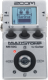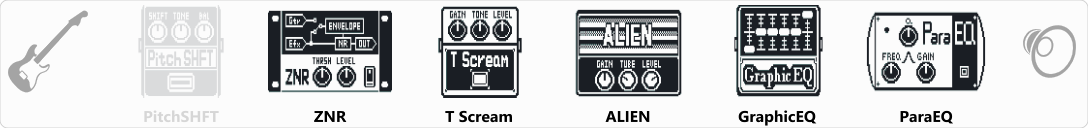# Metalcore

Discussion in 'Zoom MS-50G' started by Juan Esteban, Aug 21, 2018.

1. MetalcoreDevice: Zoom Ms-50g
Firmware: 3.00

Name on device: MetalCore
Optimized for: Guitar Amp

Effects chain:Patch for heavy metal, metalcore, etc...

Effect: "PitchSHFT" (Modulation / Sfx), active - "no"
"Shift" = -3
"Tone" = 10
"Bal" = 100
"Fine" = 0
"Level" = 100

Effect: "ZNR" (Dynamics / Filter), active - "yes"
"THRSH" = 15
"DETCT" = GtrIn
"Level" = 100

Effect: "T Scream" (Overdrive / Distortion), active - "yes"
"Gain" = 15
"Tone" = 100
"Level" = 120

Effect: "ALIEN" (Amp simulator), active - "yes"
"Gain" = 40
"Tube" = 35
"Level" = 100
"Trebl" = 75
"Middl" = 75
"Bass" = 35
"Prese" = 100
"CAB" = FD COMBO 2x12
"OUT" = COMBO POWER AMP

Effect: "GraphicEQ" (Dynamics / Filter), active - "yes"
"160Hz" = -2
"400Hz" = -3
"800Hz" = -4
"3.2kHz" = 1
"6.4kHz" = -3
"12kHz" = -3
"Level" = 100

Effect: "ParaEQ" (Dynamics / Filter), active - "yes"
"Freq1" = 800Hz
"Q1" = 1
"Gain1" = -5
"Freq2" = 400Hz
"Q2" = 1
"Gain2" = -3
"Level" = 100

Note: This is a patch file, you will need to download and install the ToneLib software to use the patch.

#### Attached Files:

• ###### Metalcore.ms50g
File size:
430 bytes
Views:
274
2. Sounds great!